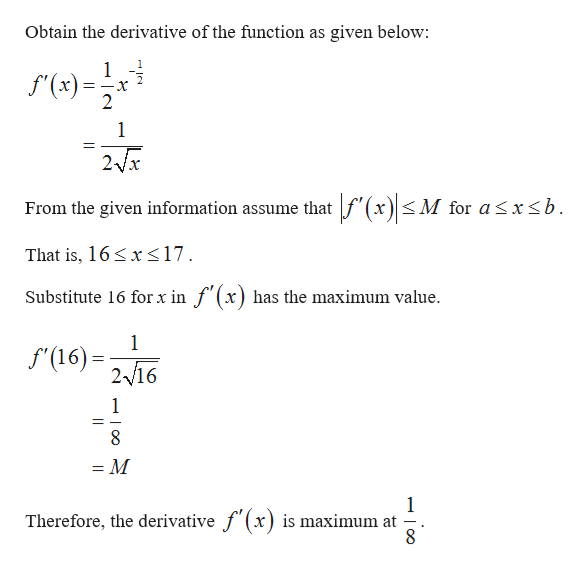# Assume that f ' (x)≤ M for a ≤ x ≤ b. One can show by the Mean ValueTheorem that f (b) − f (a) ≤ M (b − a), and thus f (a) − M(b − a) ≤ f(b) ≤ f (a) + M(b − a). Using this fact, determine a lower bound and an upper bound for 17^1/2 (Hint: Let f (x) = x^1/2, a = 16, and b = 17, and then find bounds for f (17) = 17^1/2.First, find a reasonable value for M:      M =  Then fill in the blanks:   ≤ f (17) ≤

Question
1 views

Assume that f ' (x)≤ M for a ≤ x ≤ b. One can show by the Mean Value
Theorem that f (b) − f (a) ≤ M (b − a), and thus f (a) − M(b − a) ≤ f(b) ≤ f (a) + M(b − a). Using this fact, determine a lower bound and an upper bound for 17^1/2

(Hint: Let f (x) = x^1/2, a = 16, and b = 17, and then find bounds for f (17) = 17^1/2.

First, find a reasonable value for M:      M =
Then fill in the blanks:   ≤ f (17) ≤

check_circle

Step 1

From the given information, f(x) = x1/2, a = 16 and b = 17, t...help_outlineImage TranscriptioncloseObtain the derivative of the function as given below 1 f(x)= 2 1 2/x M for asx

### Want to see the full answer?

See Solution

#### Want to see this answer and more?

Solutions are written by subject experts who are available 24/7. Questions are typically answered within 1 hour.*

See Solution
*Response times may vary by subject and question.
Tagged in

### Other